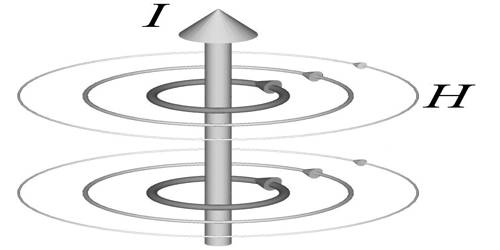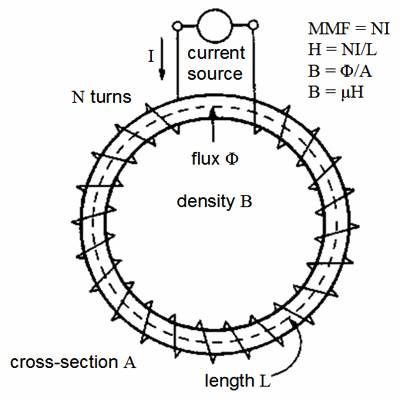Physics

# Magnetic Field IntensityMagnetic field intensity: The ratio of the magnetic induction or magnetic field to the magnetic permeability at a point in a magnetic field is called magnetic field intensity. Electric intensity the magnitude of an electric field at a point in the field, equivalent to the force that would be exerted on a little unit charge placed at the point.The magnetic fields generated by currents and calculated from Ampere’s Law or the Biot-Savart Law is characterized by the magnetic field B measured in Tesla. But when the generated fields pass through magnetic materials which themselves contribute internal magnetic fields, ambiguities can arise about what part of the field comes from the external currents and what comes from the material itself. It is denoted by H. Technically, a distinction is made between magnetic field strength H, measured in amperes per meter (A/m), and magnetic flux density B, measured in Newton-meters per ampere (Nm/A), also called teslas (T).

H = B00